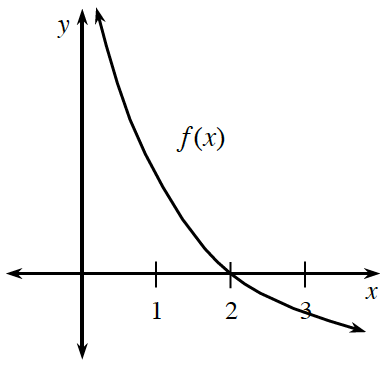### Home > APCALC > Chapter 5 > Lesson 5.2.5 > Problem5-108

5-108.

Put the following function values in order from least to greatest given the graph of $y = f\left(x\right)$ below.

$f(2), f ^\prime(2), f^{\prime \prime}(2)$Is $f\left(2\right)$ positive, negative or $0$?

Is $f^\prime\left(2\right)$ positive, negative or $0$? Recall that $f^\prime\left(x\right)$ represents the slope of $f\left(x\right)$.

Is $f^{\prime \prime}\left(2\right)$ positive, negative or $0$? Recall that $f^{\prime \prime}\left(x\right)$ represents the concavity of $f\left(x\right)$.